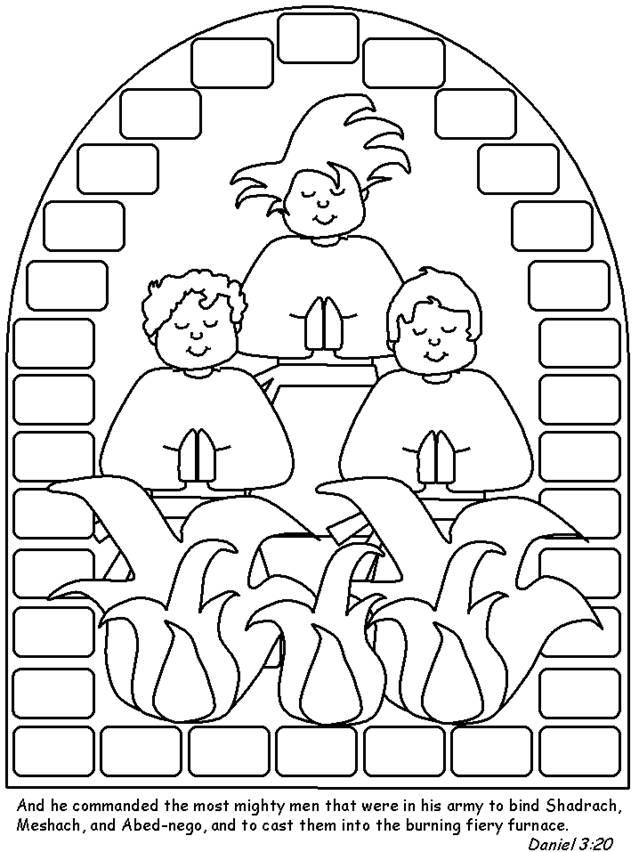# Dr. Pat's Orthodox Super Sunday School Curriculum

THE THREE YOUTHS IN THE FIRE

Home Activities:

• No, don’t burn your kids at the stake as an illustration!
• If you have the icon, put it in your icon corner.
• Try the healthy meals of the king’s house for a week – vegetables, fruit – no rich foods or meat.
• Visit the city incinerator – not your average field trip but gives the children a feel for the size of a “furnace”.
• Or visit the fire station and learn about fire safety.
• Puzzle and coloring sheet.
• Read the story at bedtime.

Prayer: Lord, let us praise You as did the three Holy Youths.

# The Three Youths in the Fire

`O U R G O D W H H S O E R N M `
`M N O L Y B A B H W V A E E E `
`S E L R V I E A I I Z B E H L `
`S E A E N B D L T Z U E C A A `
`T U O A A R D P A C E L A I S `
`I T N A A H A H H V E R N R U `
`U A S C B C S A L F R O R A R `
`H T H M T E D I H E E F U Z E `
`U S R N T N D O M A I C F A J `
`E O F L E B L N G A Z N I N G `
`F I E Z R E D C E F L B A V T `
`O B Z V L J R W Q G O E F D Q `
`N A H C A H S E M G O N X R Y `
`R H Y K K I E B S Z U Y O L V `
`Z Z J D J O Z N W K Q H Q S Y `

`ABEDNEGO               JERUSALEM`
`AZARIAH                MESHACH`
`BABYLON                MISHAEL`
`BELTESHAZZAR           NEBUCHADNEZZAR`
`CAPTIVE                SHADRACH`
`DANIEL                 SON OF GOD`
`FURNACE                STATUE`
`HANANIAH`
` `
`__ __ __   __ __ __   __ __ __ __   __ __   __ __ __ __ __   __ __   `
` `
`__ __ __ __   __ __   __ __ __ __ __ __ __   __ __   __ __ __ __   `
` `
`__ __ __   __ __ __ __ __ __ __   __ __   __ __ __ __ __ __ __   `
` `
`__ __ __ __ .`

Coloring Page: